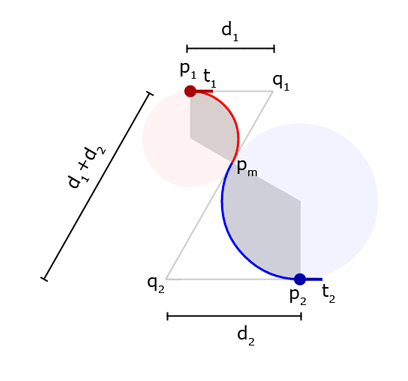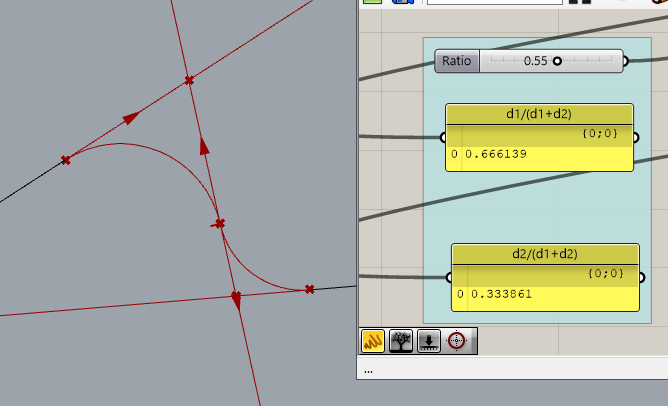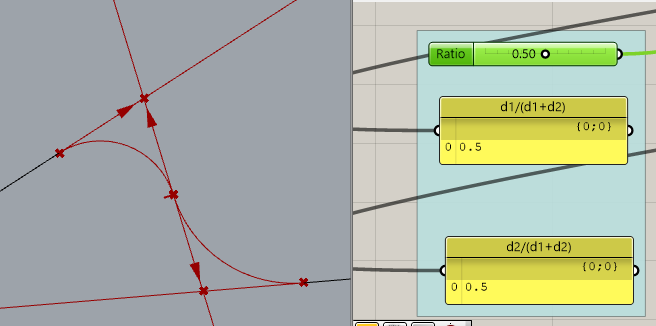# Grasshopper BiArc component ratio parameter algorithm/math?

Hi all,
We are working on architectural geometry description using the grasshopper built-in Bi-arc component, and I’m having a hard time finding the actual mathematical meaning behind the “R/ratio” parameter of this component. I’m trying to understand this because we need to be able to describe the geometry using simple math logic to the engineers for calculation and construction, so the design geometry is not a black box:

I’m assuming the bi-arc component, under the hood, is generating the bi-arc using math similar to how this article described:

My hypothesis is that R(ratio) is simply d1/(d1+d2) based on this diagram below of a typical bi-arc:But after some testing it only seems to be true when R is 0.5, but not true for any other R, see below:Does anyone know the true meaning of R and how I can re-create it geometrically in grasshopper?
@DavidRutten would really appreciate some guidance from you!
Thank you!